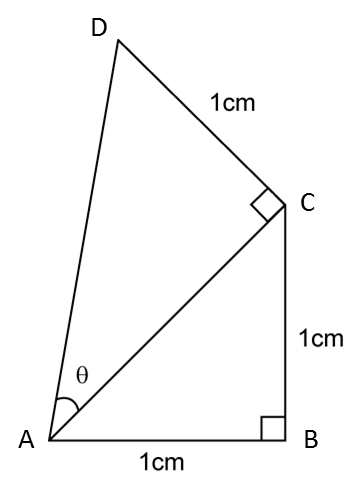# 9.3.1 Trigonometry II, SPM Paper 1 (Short Questions)

Question 1:In the diagram above, find the value of tan θ.

Solution:

$\begin{array}{l}\text{In}△\text{}ABC,\text{using Pythagoras’ Theorem,}\\ AC=\sqrt{{1}^{2}+{1}^{2}}=\sqrt{2}\text{}cm\\ \\ \mathrm{tan}\theta =\frac{CD}{AC}\\ \mathrm{tan}\theta =\frac{1}{\sqrt{2}}\end{array}$

Question 2:In the diagram above, ABCE is a rectangle and point D lies on the straight line EC. Given that DC = 5 cm and AE = 4cm, find the value of cosθ.

Solution:

Question 3:In the diagram above, PMR is a straight line, M is the midpoint of line PR. Given that QR = 12cm and sin y°= 0.6, find the value of tan x°.

Solution: